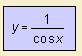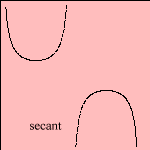# secant

## trigonometricThe secant sec(x) is defined as the divider of the cosine, the cosecant csc(x) is the divider of the sine.
The curves are equal under translation.

Derived from the secant are:
- exsecant, defined as exsec(x) = sec(x) - 1
- excosecant, defined as excsc(x) = csc(x) - 1

The inverse functions of the secant and the cosecant are called the arc secant arcsec(x) and the arc cosecant arccsc(x), respectively. These are cyclometric functions.